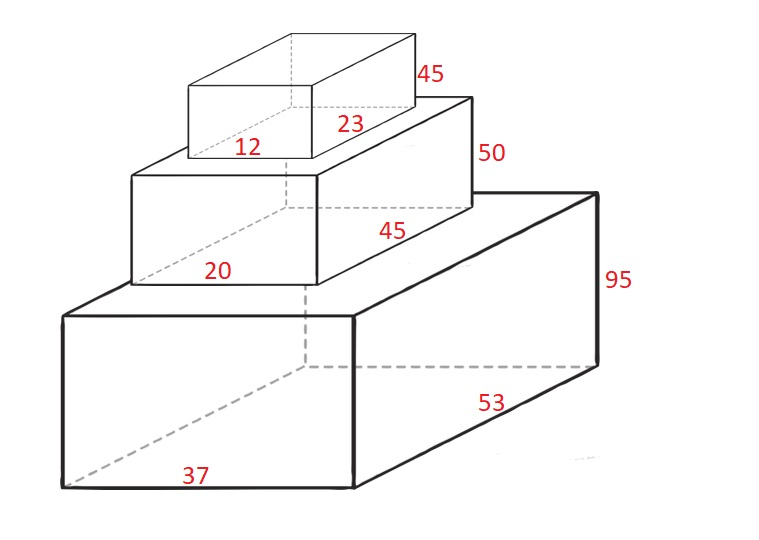1691. Maximum Height by Stacking Cuboids

Given `n` `cuboids` where the dimensions of the `ith` cuboid is `cuboids[i] = [widthi, lengthi, heighti]` (0-indexed). Choose a subset of `cuboids` and place them on each other.

You can place cuboid `i` on cuboid `j` if `widthi <= widthj` and `lengthi <= lengthj` and `heighti <= heightj`. You can rearrange any cuboid's dimensions by rotating it to put it on another cuboid.

Return the maximum height of the stacked `cuboids`.

Example 1:```Input: cuboids = [[50,45,20],[95,37,53],[45,23,12]]
Output: 190
Explanation:
Cuboid 1 is placed on the bottom with the 53x37 side facing down with height 95.
Cuboid 0 is placed next with the 45x20 side facing down with height 50.
Cuboid 2 is placed next with the 23x12 side facing down with height 45.
The total height is 95 + 50 + 45 = 190.
```

Example 2:

```Input: cuboids = [[38,25,45],[76,35,3]]
Output: 76
Explanation:
You can't place any of the cuboids on the other.
We choose cuboid 1 and rotate it so that the 35x3 side is facing down and its height is 76.
```

Example 3:

```Input: cuboids = [[7,11,17],[7,17,11],[11,7,17],[11,17,7],[17,7,11],[17,11,7]]
Output: 102
Explanation:
After rearranging the cuboids, you can see that all cuboids have the same dimension.
You can place the 11x7 side down on all cuboids so their heights are 17.
The maximum height of stacked cuboids is 6 * 17 = 102.
```

Constraints:

• `n == cuboids.length`
• `1 <= n <= 100`
• `1 <= widthi, lengthi, heighti <= 100`

1691. Maximum Height by Stacking Cuboids
``````struct Solution;

impl Solution {
fn max_height(mut cuboids: Vec<Vec<i32>>) -> i32 {
let n = cuboids.len();
for i in 0..n {
cuboids[i].sort_unstable();
}
cuboids.sort_unstable();
let mut dp: Vec<i32> = vec![0; n];
for i in 0..n {
dp[i] = cuboids[i];
'outer: for j in 0..i {
for k in 0..3 {
if cuboids[j][k] > cuboids[i][k] {
continue 'outer;
}
}
dp[i] = dp[i].max(dp[j] + cuboids[i]);
}
}
dp.into_iter().max().unwrap()
}
}

#[test]
fn test() {
let cuboids = vec_vec_i32![[50, 45, 20], [95, 37, 53], [45, 23, 12]];
let res = 190;
assert_eq!(Solution::max_height(cuboids), res);
let cuboids = vec_vec_i32![[38, 25, 45], [76, 35, 3]];
let res = 76;
assert_eq!(Solution::max_height(cuboids), res);
let cuboids = vec_vec_i32![
[7, 11, 17],
[7, 17, 11],
[11, 7, 17],
[11, 17, 7],
[17, 7, 11],
[17, 11, 7]
];
let res = 102;
assert_eq!(Solution::max_height(cuboids), res);
}
``````